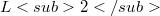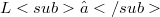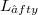﻿

### On the estimate the$L2$ and$L∞$ norm of the error for the initial value problems

#### Abstract

Our objective in this paper is to examine conditions under which the variational principle has a global extremal properties and to complete the error estimate procedure of .We will use the value of the functional to estimate the$L2$ and$L_∈fty$ norm of the error for the initial value problems, described by a non-linear second order differential equation belonging to the class treated in . In our analysis integral inequalities given in [1-4] are of central importance.The analysis will be illustrated by an concrete example.

DOI Code: 10.1285/i15900932v15n1p121

Full Text: PDF